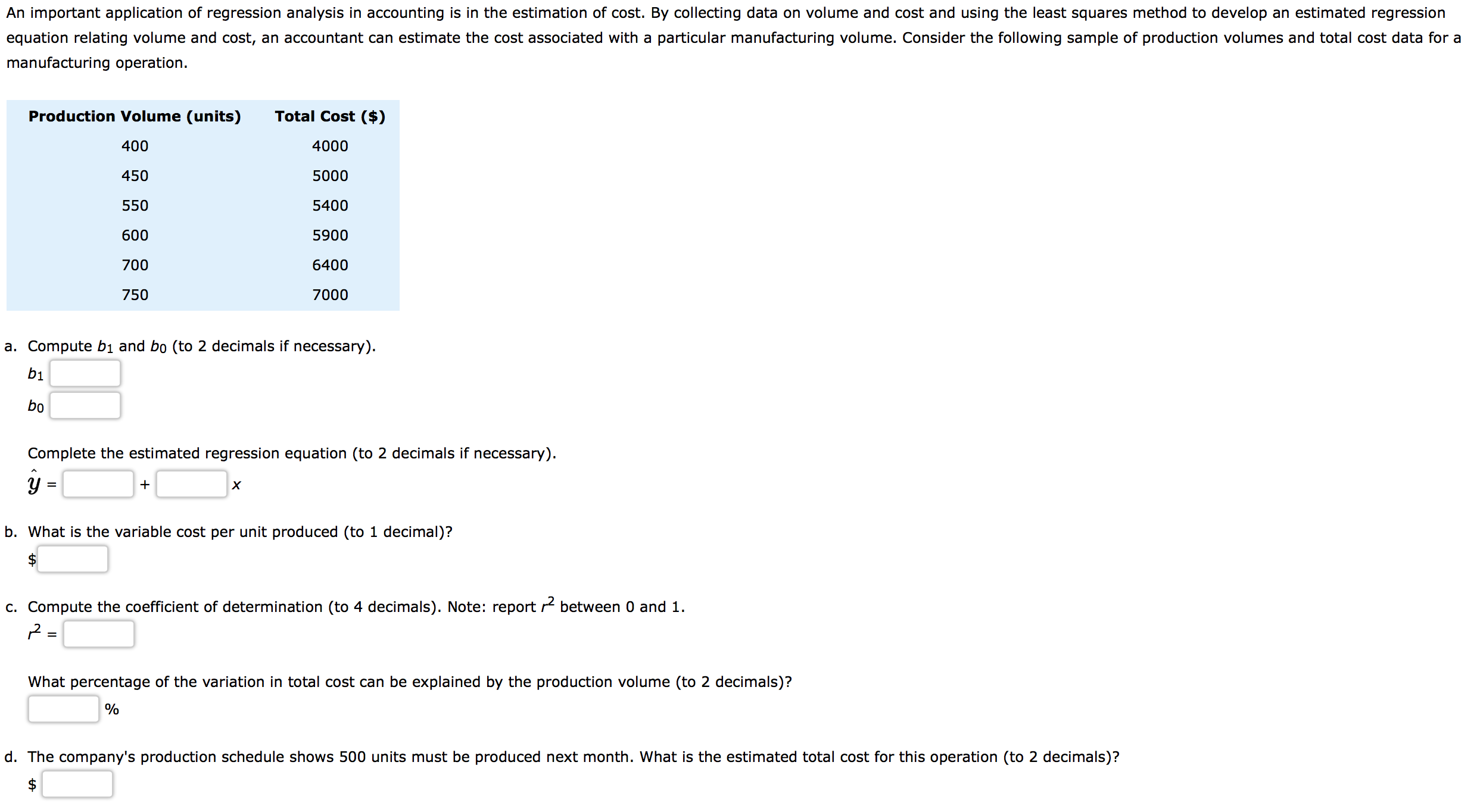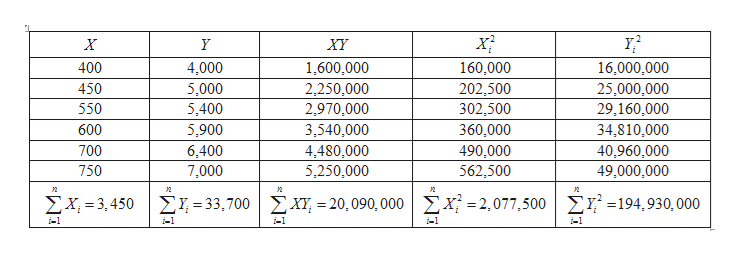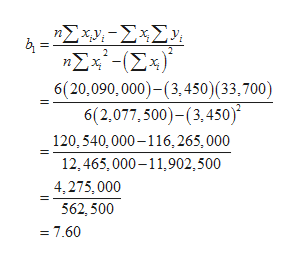# An important application of regression analysis in accounting is in the estimation of cost. By collecting data on volume and cost and using the least squares method to develop an estimated regressionequation relating volume and cost, an accountant can estimate the cost associated with a particular manufacturing volume. Consider the following sample of production volumes and total cost data for amanufacturing operation.Production Volume (units)Total Cost (\$)400400045050005505400590060070064007000750a. Compute bị and bo (to 2 decimals if necessary).bịboComplete the estimated regression equation (to 2 decimals if necessary).хb. What is the variable cost per unit produced (to 1 decimal)?c. Compute the coefficient of determination (to 4 decimals). Note: report r between 0 and 1.=What percentage of the variation in total cost can be explained by the production volume (to 2 decimals)?d. The company's production schedule shows 500 units must be produced next month. What is the estimated total cost for this operation (to 2 decimals)?

Question
22 viewshelp_outlineImage TranscriptioncloseAn important application of regression analysis in accounting is in the estimation of cost. By collecting data on volume and cost and using the least squares method to develop an estimated regression equation relating volume and cost, an accountant can estimate the cost associated with a particular manufacturing volume. Consider the following sample of production volumes and total cost data for a manufacturing operation. Production Volume (units) Total Cost (\$) 400 4000 450 5000 550 5400 5900 600 700 6400 7000 750 a. Compute bị and bo (to 2 decimals if necessary). bị bo Complete the estimated regression equation (to 2 decimals if necessary). х b. What is the variable cost per unit produced (to 1 decimal)? c. Compute the coefficient of determination (to 4 decimals). Note: report r between 0 and 1. = What percentage of the variation in total cost can be explained by the production volume (to 2 decimals)? d. The company's production schedule shows 500 units must be produced next month. What is the estimated total cost for this operation (to 2 decimals)? fullscreen
check_circle

Step 1

a.

Let X be the production volume and Y be the total cost

From the given datahelp_outlineImage TranscriptioncloseY? XY 400 4,000 1,600,000 160,000 16,000,000 450 5,000 5,400 2,250,000 202,500 302,500 25,000,000 550 2,970,000 29,160,000 600 5,900 3,540,000 360,000 34,810,000 700 6,400 4,480,000 5,250,000 490,000 40,960,000 750 7,000 562,500 49,000,000 EX, = 3,450 ΣΥ-3,700 | ΣΧ- 20, 090, 000 |ΣΧ2077,500| ΣΥ -194, 930, 000 %3D i-1 i-1 i-1 i-1 i-1 fullscreen
Step 2

The value of slope (b1) ishelp_outlineImage TranscriptioncloseΣχν- Σ<Σ) 4 = ηΣx- (Σx)" 6(20,090, 000)-(3,450)(33,700) 6(2,077, 500)-(3, 450) 120, 540, 000 –116, 265,000 12, 465, 000–11,902,500 4,275, 000 562, 500 = 7.60 fullscreen
Step 3

The value of slope (b...

### Want to see the full answer?

See Solution

#### Want to see this answer and more?

Solutions are written by subject experts who are available 24/7. Questions are typically answered within 1 hour.*

See Solution
*Response times may vary by subject and question.
Tagged in

### Other